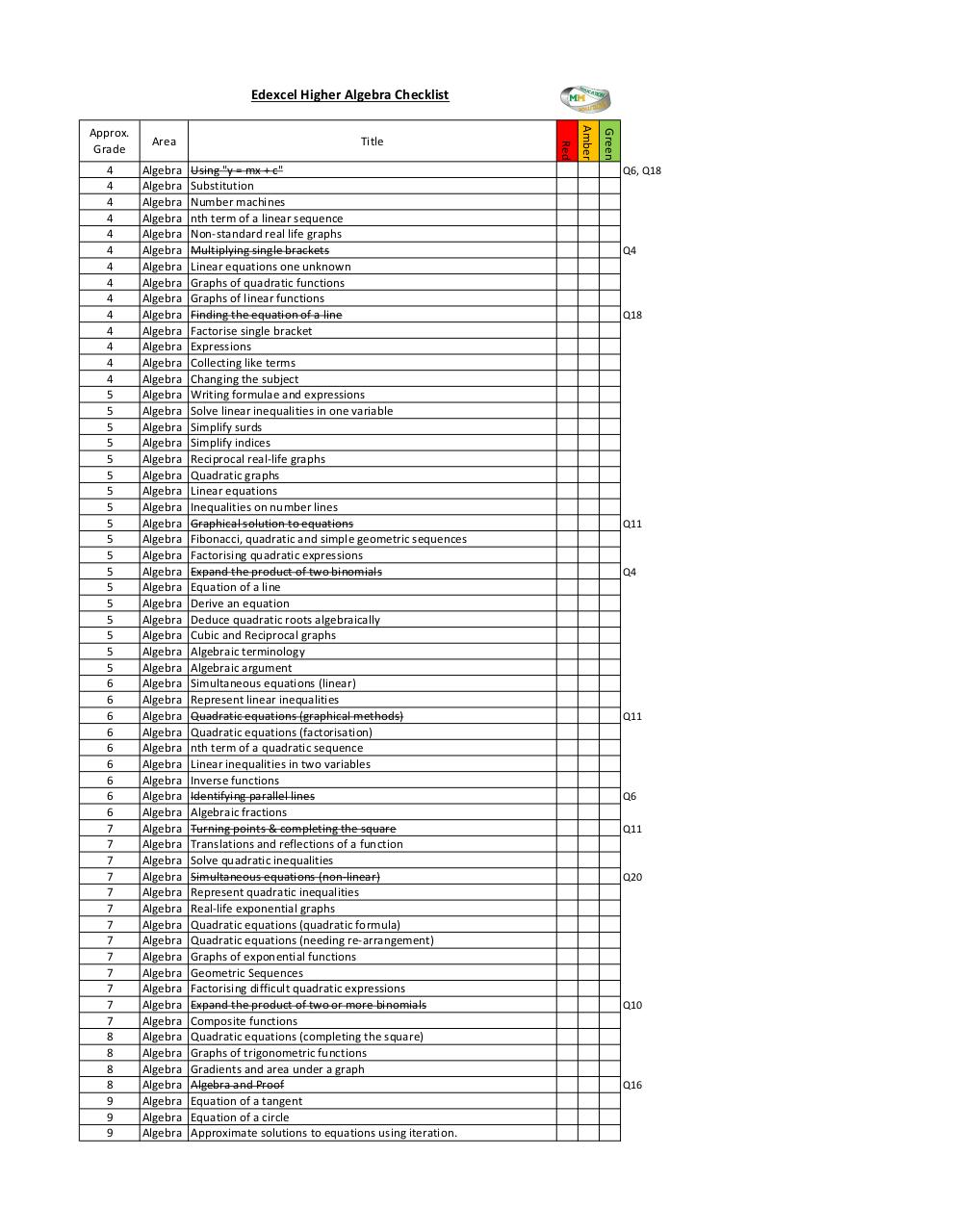# Algebra (Post Paper 1) .pdf

### File information

Original filename: Algebra (Post Paper 1).pdf
Title: Checklist (Post Paper 1).xlsx
Author: Michael

This PDF 1.7 document has been generated by / Microsoft: Print To PDF, and has been sent on pdf-archive.com on 25/05/2017 at 16:01, from IP address 188.222.x.x. The current document download page has been viewed 374 times.
File size: 344 KB (1 page).
Privacy: public file

Algebra (Post Paper 1).pdf (PDF, 344 KB)

### Document preview

Edexcel Higher Algebra Checklist

Algebra
Algebra
Algebra
Algebra
Algebra
Algebra
Algebra
Algebra
Algebra
Algebra
Algebra
Algebra
Algebra
Algebra
Algebra
Algebra
Algebra
Algebra
Algebra
Algebra
Algebra
Algebra
Algebra
Algebra
Algebra
Algebra
Algebra
Algebra
Algebra
Algebra
Algebra
Algebra
Algebra
Algebra
Algebra
Algebra
Algebra
Algebra
Algebra
Algebra
Algebra
Algebra
Algebra
Algebra
Algebra
Algebra
Algebra
Algebra
Algebra
Algebra
Algebra
Algebra
Algebra
Algebra
Algebra
Algebra
Algebra
Algebra
Algebra
Algebra
Algebra

Using "y = mx + c"
Substitution
Number machines
nth term of a linear sequence
Non-standard real life graphs
Multiplying single brackets
Linear equations one unknown
Graphs of linear functions
Finding the equation of a line
Factorise single bracket
Expressions
Collecting like terms
Changing the subject
Writing formulae and expressions
Solve linear inequalities in one variable
Simplify surds
Simplify indices
Reciprocal real-life graphs
Linear equations
Inequalities on number lines
Graphical solution to equations
Fibonacci, quadratic and simple geometric sequences
Expand the product of two binomials
Equation of a line
Derive an equation
Cubic and Reciprocal graphs
Algebraic terminology
Algebraic argument
Simultaneous equations (linear)
Represent linear inequalities
nth term of a quadratic sequence
Linear inequalities in two variables
Inverse functions
Identifying parallel lines
Algebraic fractions
Turning points &amp; completing the square
Translations and reflections of a function
Simultaneous equations (non-linear)
Real-life exponential graphs
Graphs of exponential functions
Geometric Sequences
Expand the product of two or more binomials
Composite functions
Graphs of trigonometric functions
Gradients and area under a graph
Algebra and Proof
Equation of a tangent
Equation of a circle
Approximate solutions to equations using iteration.

Green

4
4
4
4
4
4
4
4
4
4
4
4
4
4
5
5
5
5
5
5
5
5
5
5
5
5
5
5
5
5
5
5
6
6
6
6
6
6
6
6
6
7
7
7
7
7
7
7
7
7
7
7
7
7
8
8
8
8
9
9
9

Title

Red

Area

Amber

Approx.

Q6, Q18

Q4

Q18

Q11

Q4

Q11

Q6
Q11

Q20

Q10

Q16#### HTML Code

Copy the following HTML code to share your document on a Website or Blog

#### QR Code### Related keywords